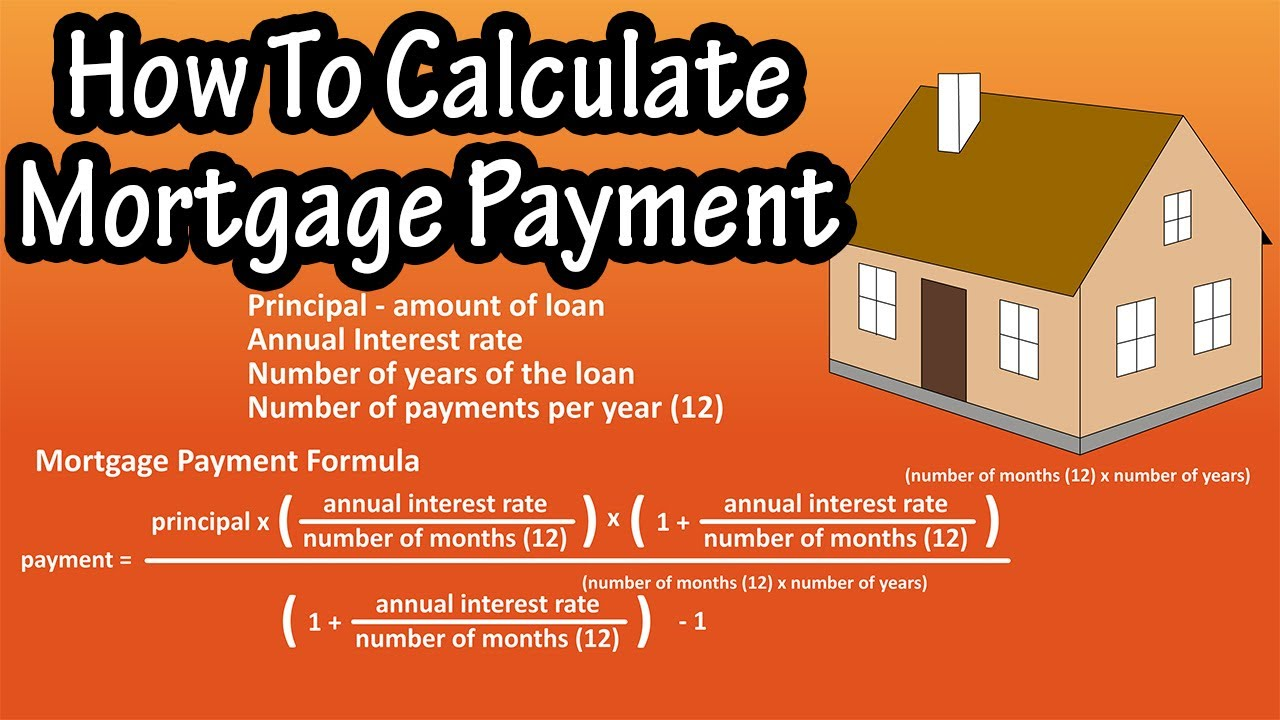In this video we discuss how to calculate a mortgage payment. We go through the formula for calculating a mortgage payment step by step and cover the numbers you need to know to do the calculation. We also show the true total cost over the life of the home loan.

Transcript/notes
To calculate a mortgage payment for a fixed rate mortgage, where the interest rate never changes, there are 4 things you need to know, the principal, or the amount owed after the down payment, the annual interest rate, the number of total years for the loan, and the number of payments per year, which typically is once a month, so 12 payments per year.

The formula for calculating a mortgage payment is the principal, times the quantity, annual percentage rate divided by the number of months in a year, times the quantity 1 plus annual percentage rate divided by the number of months in a year, with this quantity raised to the number of months times the total number of years of the loan, divided by the quantity 1 plus annual percentage rate divided by the number of months in a year, raised to the number of months times the number of years, minus 1.

And I have shortened the formula using the symbols as you see here.
This formula may seem a bit intimidating, but if you break it down, it is much easier to work with.

So, lets say that after your down payment, you are left owing \$200,000, which is your principal. The annual interest rate is 4.5%, the loan is for 30 years, and there are 12 payments per year.

In our formula, we need to know the annual percentage rate divided by the number of months in a year. Our annual rate is 4.5%, which mathematically is 0.045, and the number of months is 12, so 0.045 divided by 12, which calculates out to 0.00375.

Next in the formula we need to know 1 plus annual percentage rate divided by the number of months in a year, we just calculated the annual percentage rate divided by the number of months in a year, so this is 1 plus 0.00375, which is 1.00375.

Next we need to calculate this raised value of the number of months times the number of years, which is 12 payments per year times 30 years, which is 360.

Next, we can now calculate this whole value of 1 plus annual percentage rate divided by the number of months in a year, raised to the number of months times the number of years. So, we have 1.00375 raised to 360.
If you recall, when we raise some number to a power, such as 2 raised to the 3, we just multiply that number times itself that many times. So, 2 times 2 times 2 times 2, which is 8.

In our formula this is 1.00375 times itself 360 times. Using a calculator or excel, you would type in equals 1.00375 ^, which is shift 6 on a keyboard, 360. And the result is 3.847699 rounding off.

Now, we have everything in basic terms to plug and calculate into our formula. So, \$200,000 times 0.00375, times 3.847699, divided by 3.847699 minus 1. And this calculates out to 1013.37 rounding off, and that would be the monthly mortgage payment.

There are also mortgage calculators online, and you can see here that using the numbers, we get the same result.

One thing to take note of is the total cost of the house, which is 1013.37 times 360, the number of payments, and this gives us \$364,813.20. If the interest rate was 4.0%, this would result in a monthly payment of \$957.83 and a total cost of \$343,739, so \$21,074.20 less.

And this is why the interest rate on any loan is so important to you as a buyer.

Timestamps
0:00 4 things needed to calculate a mortgage payment
0:19 Formula for calculating mortgage payments
0:56 Example mortgage payment calculation
3:00 Final payment calculated out
3:14 Total cost of the loan
3:27 Comparing interest rates

source